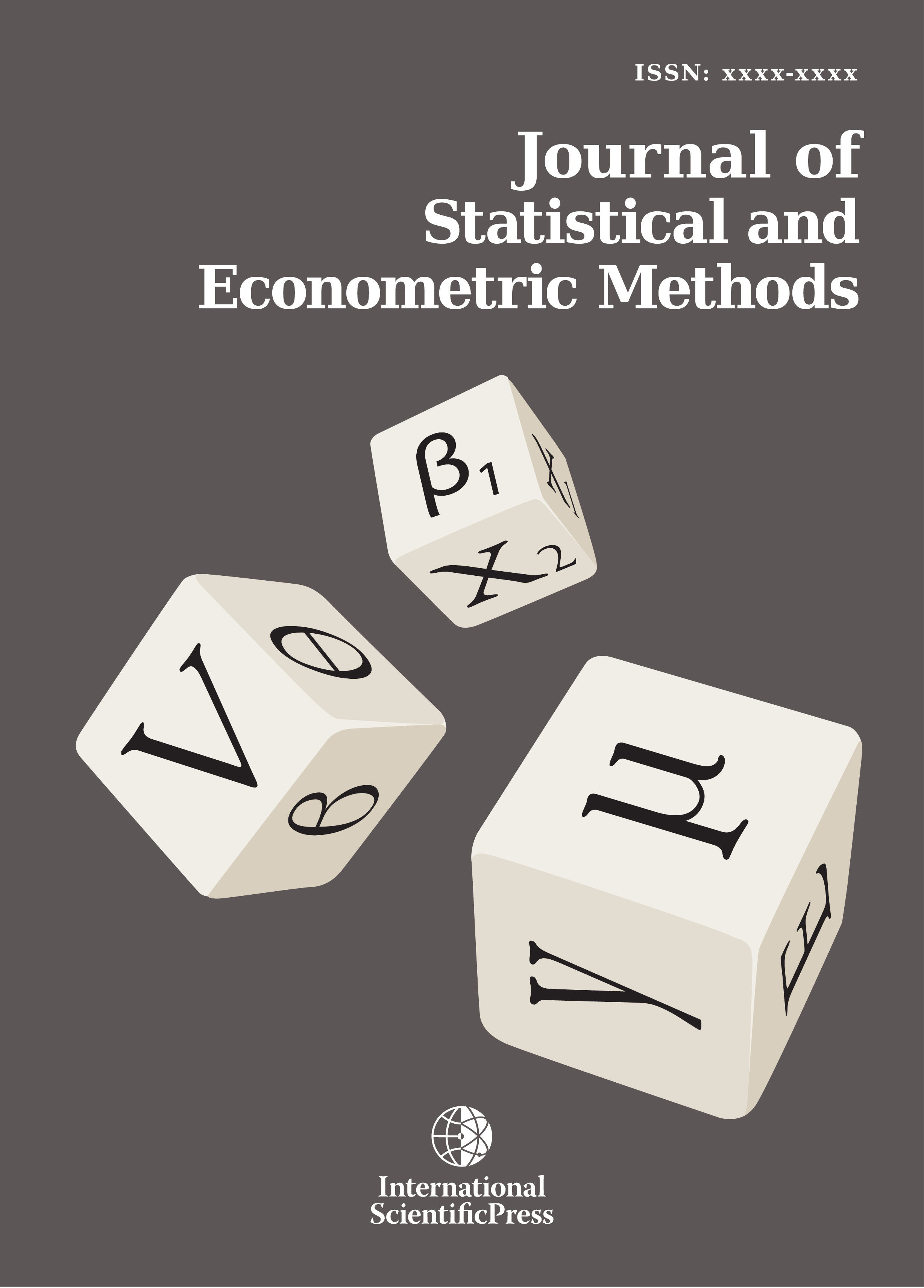# Journal of Statistical and Econometric Methods

#### Estimation of Gini coefficients using Lorenz curves

•[ Download ]
• Times downloaded: 3179
• Abstract

Primary income data yields the most exact estimates of the Gini coefficient. Using Lorenz curves, the Gini coefficient is defined as the ratio of the area between the diagonal and the Lorenz curve and the area of the whole triangle under the diagonal. Various attempts have been made to obtain accurate estimates. The trapezium rule is simple, but yields a positive bias for the area under the Lorenz curve and, consequently, a negative bias for the Gini coefficient. Simpson´s rule is better fitted to the Lorenz curve, but this rule demands an even number of subintervals of the same length. Lagrange polynomials of second degree can be considered as a generalisation of Simpson´s rule because they do not demand equidistant points. If the subintervals are of the same length, the Lagrange polynomial method is identical with Simpson´s rule. In this study, we compare different methods. When we apply Simpson´s rule, we mainly consider Lorenz curves with deciles. In addition, we use the trapezium rule, Lagrange polynomials and generalizations of Golden´s method (2008). No method is uniformly optimal, but the trapezium rule is almost always inferior and Simpson´s rule is superior. Golden´s method is usually of medium quality.ISSN: 2241-0376 (Online)
2241-0384 (Print)